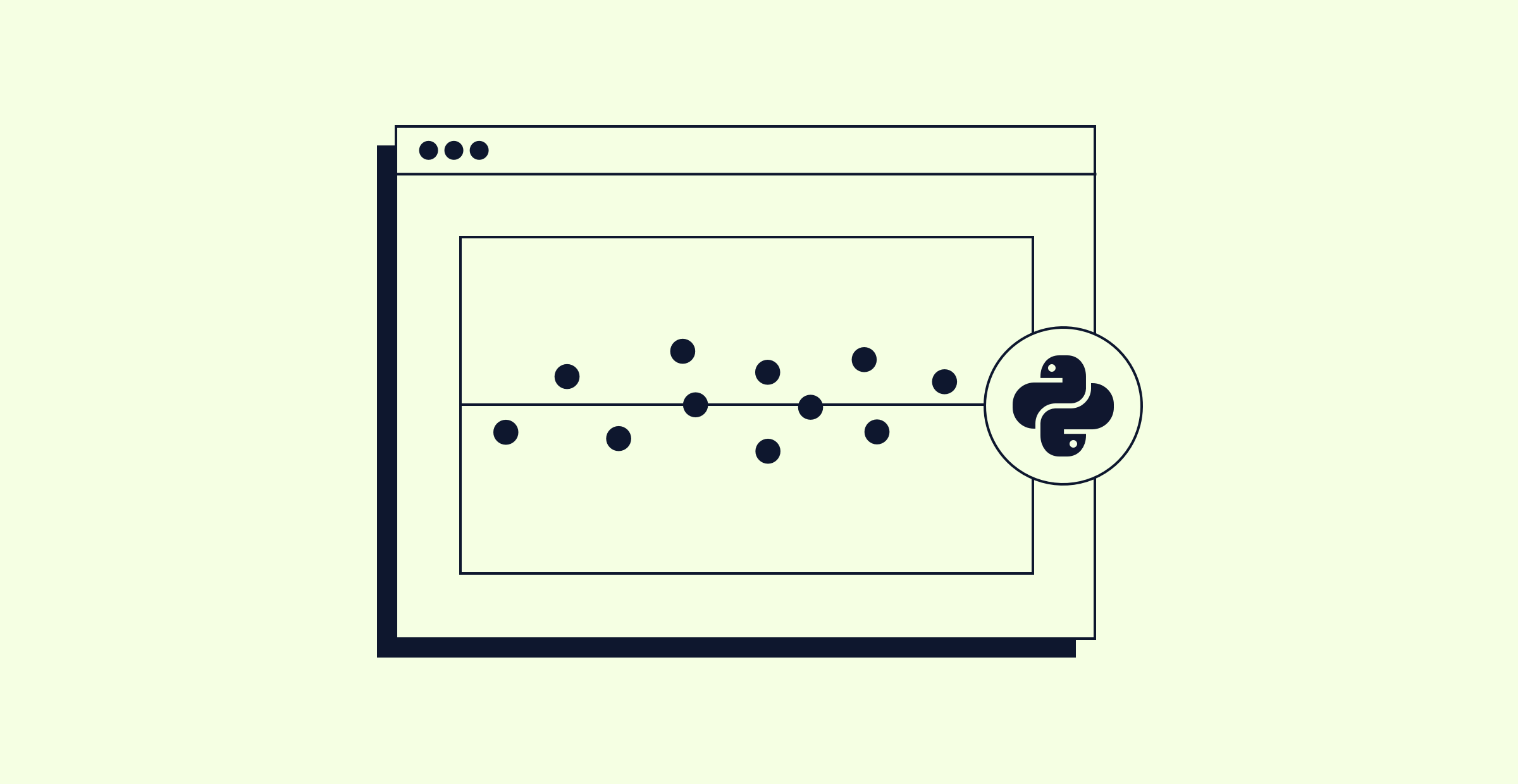# Level Up: Linear Regression in Python - Part 4In the fourth lesson of the series, we'll talk about the matrix representation of the linear regression problem. In the process, we'll discuss the basics of matrix multiplication. We'll also see how this mathematical understanding can prepare us to make sense of error messages that we might encounter when fitting a model in Python.

Here are some Stack Overflow questions related to the work we did in today's session:

If you want to ask any questions or provide feedback on the lesson, you are welcome to leave a comment on the YouTube recording of this lesson. If you’d like to watch a session live, follow the Codecademy YouTube channel. We'll be live again on Tuesday, June 15 at 11am EDT to discuss polynomial and interaction terms, which can be used to build more flexible regression models. You can join that session here.

Finally, if you want even more linear regression content, you can sign up for the Linear Regression in Python interactive course this series was based on. This course was developed by Sophie and has many more quizzes, projects, and helpful nuggets that we can’t fit into our streams!